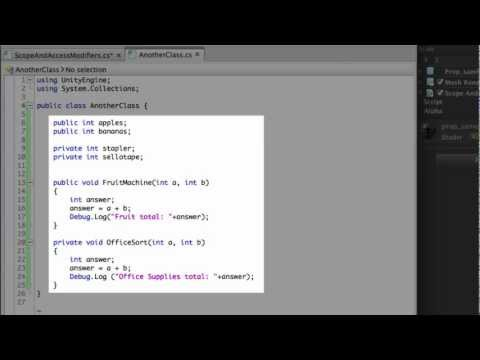Scope and Access Modifiers

-

Understanding variable & function scope and accessibility.Scope and Access Modifiers

Principiante Scripting

Code snippet

using UnityEngine;
using System.Collections;

public class ScopeAndAccessModifiers : MonoBehaviour
{
public int alpha = 5;

private int beta = 0;
private int gamma = 5;

private AnotherClass myOtherClass;

void Start ()
{
alpha = 29;

myOtherClass = new AnotherClass();
myOtherClass.FruitMachine(alpha, myOtherClass.apples);
}

void Example (int pens, int crayons)
{
answer = pens * crayons * alpha;
}

void Update ()
{
Debug.Log("Alpha is set to: " + alpha);
}
}
#pragma strict

public var alpha : int = 5;

private var beta : int = 0;
private var gamma : int = 5;

private var myOtherClass : AnotherClass;

function Start ()
{
alpha = 29;

myOtherClass = new AnotherClass();
myOtherClass.FruitMachine(alpha, myOtherClass.apples);
}

function Example (pens : int, crayons : int)
{
var answer : int;
answer = pens * crayons * alpha;
}

function Update ()
{
Debug.Log("Alpha is set to: " + alpha);
}
import UnityEngine
import System.Collections

public class ScopeAndAccessModifiers(MonoBehaviour):

public alpha = 5

private beta = 0

private gamma = 5

private myOtherClass as AnotherClass

private def Start():
alpha = 29

myOtherClass = AnotherClass()
myOtherClass.FruitMachine(alpha, myOtherClass.apples)

private def Example(pens as int, crayons as int):
answer = ((pens * crayons) * alpha)

private def Update():
Debug.Log(('Alpha is set to: ' + alpha))

Code snippet

using UnityEngine;
using System.Collections;

public class AnotherClass
{
public int apples;
public int bananas;

private int stapler;
private int sellotape;

public void FruitMachine (int a, int b)
{
answer = a + b;
Debug.Log("Fruit total: " + answer);
}

private void OfficeSort (int a, int b)
{
answer = a + b;
Debug.Log("Office Supplies total: " + answer);
}
}
#pragma strict

public var apples : int;
public var bananas : int;

private var stapler : int;
private var sellotape : int;

public function FruitMachine (a : int, b : int)
{
var answer : int;
answer = a + b;
Debug.Log("Fruit total: " + answer);
}

private function OfficeSort (a : int, b : int)
{
var answer : int;
answer = a + b;
Debug.Log("Office Supplies total: " + answer);
}
import UnityEngine
import System.Collections

public class AnotherClass:

public apples as int

public bananas as int

private stapler as int

private sellotape as int

public def FruitMachine(a as int, b as int):